# How to Subtract in Google Sheets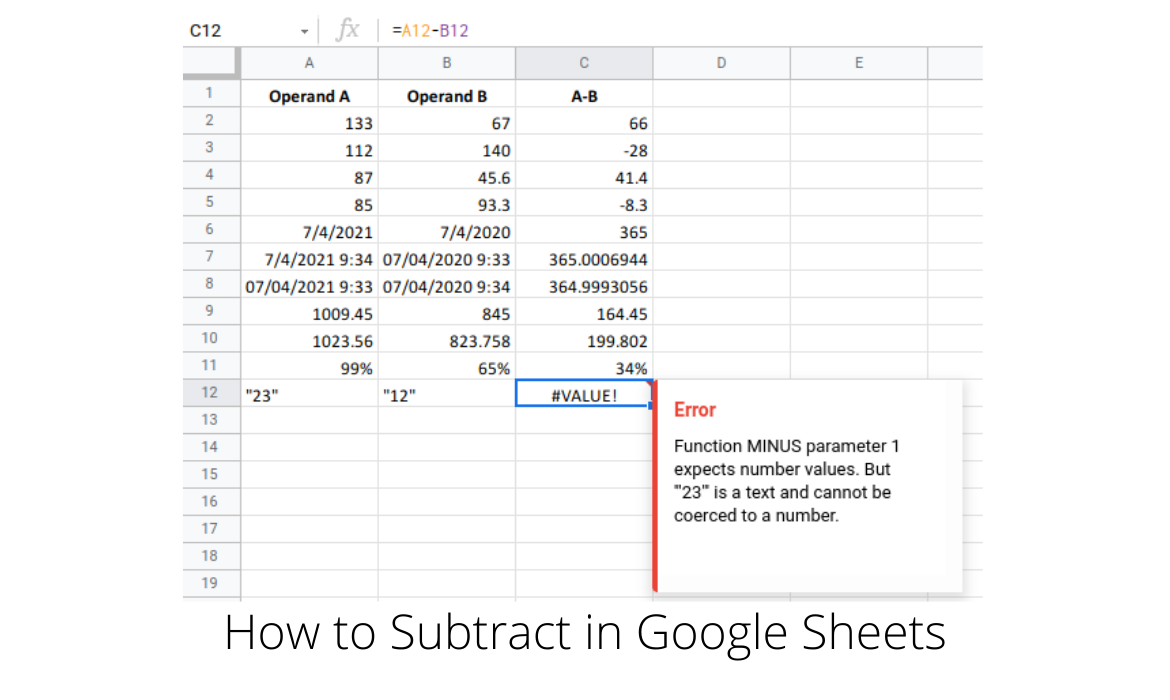Mathematical operations are among the most fundamental functions available to any computer program.

For spreadsheets, they are the building blocks for making among other things, financial calculations.

The most basic mathematical operations are addition, subtraction, multiplication, and division.Since subtraction is a mathematical operation, it expects its operands to be numeric.

In Google sheets, this means it can be any type found under the Format > Number option.

This covers integers, decimal numbers, percentages, currency amounts, dates and time.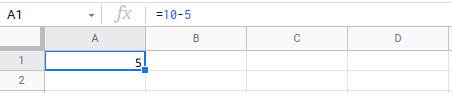The simplest way to perform a subtraction is to enter the operation directly into a cell, such as the one shown in the example above where we are subtracting 5 from 10.

It is important to note that in order for Google sheets to process this as a mathematical operation, it must start with an = (equals) sign, and so we enter =10-5.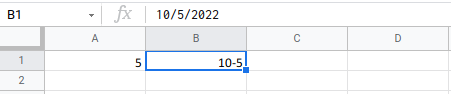If we omit the equals sign, Google sheets will attempt a best guess at the type of the entered data, and in this case results in a date type with value 10-5-2022, as seen above.

Returning to our original formula of =10-5, while Google sheets will happily evaluate this and any other similar operations, it is not very practical to directly enter operands in this manner.

It is more customary to use values contained in cells, and reference those cells in formulas.To demonstrate this, we’ll use a very simple sheet that contains two columns, titled Operand A and Operand B, which contain numeric values of different numeric types, as well as text values in the last row.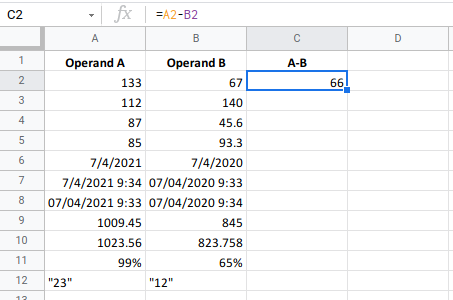In column C, we will show the results of subtracting the contents of the values of Operand B from Operand A for each row.

The formula used for the first row is =A2-B2, and the result of 66 is shown in cell C2.

We can apply the formula in cell C2 to the remainder of the cells in column C quite easily and quickly using the fill handle, as shown above. Google sheets will automatically adjust the cell references in the formula.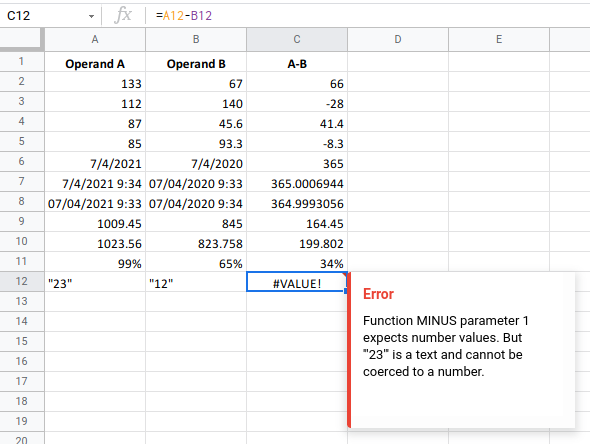Applying the corresponding formula to the remainder of the rows, produces the above results.

There are a few things worth noting here. Firstly, notice that rows 6 to 8 with date and datetime values, produce valid results.

The difference between two dates or two datetimes, produces a result in days and fractions of days (expressed as a decimal value).

Also notice that subtracting percentages is easily handled by Google sheets, and it too produces valid results.

As expected, subtracting the text values in row 12 generates an error message.

It is interesting to look at the error message, which refers to the function MINUS, even though we have not used such a function in any of our formulas.

Even though we’ve directly entered the operation into column C, Google sheets converts this into a function call.

This leads us nicely into another way of performing subtraction in Google sheets, with the function MINUS.

The definition of the MINUS function is as follows:

## MINUS function

Returns the difference of two numbers. Equivalent to the `-` operator.

MINUS(A2,A3)

MINUS(3,4)

### Syntax

MINUS(value1, value2)

• value1 – The minuend, or number to be subtracted from.
• value2 – The subtrahend, or number to subtract from value1.Hence, an equivalent to using the simple =A2-B2 formula, is to use the MINUS function, which as its syntax shows, takes two parameters.

The function is implemented in the formula in column D, =MINUS(A2,B2), and subtracts the contents of cell B2 from those in cell A2.

We can use the fill handle here too, to propagate the formula to the other cells in column D.

As we can see, the results in column D are exactly the same as those in column C, including the error in row 12 where the operands are text values.One final exercise is to subtract more than one operand.

In the above sheet, column C now has a third operand. Column D has the results of subtracting Operands B and C from Operand A with the formula =A2-B2-C2.

Likewise, column E is the same formula but expressed using the MINUS function.

Since the MINUS function only takes two parameters, we need to nest one MINUS function call inside another, and so the formula becomes =MINUS(MINUS(A2,B2),C2).

Column E also uses the MINUS function but with a small difference in the ordering.

The formula here is =MINUS(A2,MINUS(B2,C2)).

This new ordering evaluates B2 – C2 first, and then subtracts that result from A2, which produces a very different result to the formulas in columns D and E.

It is similar to doing A2 – (B2 – C2).

This highlights the fact that when using brackets or the MINUS function, it is important to consider the ordering.

## Conclusion

As we’ve seen, subtraction in Google sheets is fairly straightforward.

We can create a formula using either the minus operator or the MINUS function, they are both equivalent.

We can also perform subtraction with more than two operands, although since the MINUS function only accepts two parameters, we need to use nested MINUS function calls.

When nesting MINUS function calls, we need to be aware of the ordering, since it affects the result.

Costas has a wide range of experience in Information Technology covering computer hardware, programming, telecommunications, networking, web services, and general IT support. He's worked in various roles such as PHP programmer and web developer, technical and desktop support, hardware repair, system administration. Costas has excellent background in Microsoft Windows and Office Suite (Excel, Word, Powerpoint, etc), as well as a thorough understanding of Networking and Hardware maintenance.